# Python | Pandas TimedeltaIndex.symmetric_difference()

Python is a great language for doing data analysis, primarily because of the fantastic ecosystem of data-centric python packages. Pandas is one of those packages and makes importing and analyzing data much easier.

Pandas` TimedeltaIndex.symmetric_difference()` function compute the symmetric difference of two Index objects. It’s sorted if sorting is possible. For a given pair of TimedeltaIndex object `idx1` and `idx2`, symmetric_difference contains elements that appear in either `idx1 `or `idx2 `but not both. Equivalent to the Index created by `idx1.difference(idx2)` or `idx2.difference(idx1)` with duplicates dropped.

Syntax : TimedeltaIndex.symmetric_difference(other, result_name=None)

Parameters :
other : Index or array-like
result_name : str

Return : symmetric_difference : Index

Example #1: Use `TimedeltaIndex.symmetric_difference()` function to find the symmetric difference of two TimedeltaIndex objects.

 `# importing pandas as pd ` `import` `pandas as pd ` ` `  `# Create the first TimedeltaIndex object ` `tidx1 ``=` `pd.TimedeltaIndex(data ``=``[``'06:05:01.000030'``, ``'+23:59:59.999999'``, ` `                         ``'22 day 2 min 3us 10ns'``, ``'+23:29:59.999999'``, ` `                         ``'+12:19:59.999999'``]) ` ` `  `# Create the seccond TimedeltaIndex object ` `tidx2 ``=` `pd.TimedeltaIndex(data ``=``[``'09:11:18.000030'``, ``'+23:59:59.999999'``, ` `                         ``'9 day 18 min 3us '``, ``'+23:29:59.999999'``, ` `                         ``'+12:19:59.999999'``]) ` ` `  `# Print the first TimedeltaIndex object ` `print``(tidx1) ` ` `  `# Print the second TimedeltaIndex object ` `print``(tidx2) `

Output :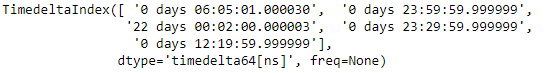Now we will use the `TimedeltaIndex.symmetric_difference()` function to find the symmetric difference.

 `# find the symmetric difference ` `tidx1.symmetric_difference(tidx2) `

Output :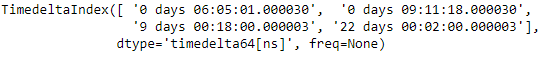As we can see in the output, the `TimedeltaIndex.symmetric_difference()` function has returned a new object which contains only those elements which are not common to both the objects.

Example #2: Use `TimedeltaIndex.symmetric_difference()` function to find the symmetric difference of two TimedeltaIndex objects.

 `# importing pandas as pd ` `import` `pandas as pd ` ` `  `# Create the first TimedeltaIndex object ` `tidx1 ``=` `pd.TimedeltaIndex(start ``=``'1 days 02:00:12.001124'``,  ` `                          ``periods ``=` `5``, freq ``=``'D'``, name ``=``'Koala'``) ` ` `  `# Create the second TimedeltaIndex object ` `tidx2 ``=` `pd.TimedeltaIndex(start ``=``'3 days 02:00:12.001124'``, ` `                          ``periods ``=` `5``, freq ``=``'D'``, name ``=``'Koala'``) ` ` `  `# Print the first TimedeltaIndex object ` `print``(tidx1) ` ` `  `# Print the second TimedeltaIndex object ` `print``(tidx2) `

Output :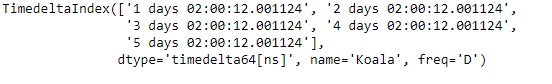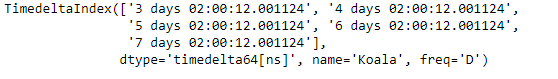Now we will use the `TimedeltaIndex.symmetric_difference()` function to find the symmetric difference.

 `# find the symmetric difference ` `tidx1.symmetric_difference(tidx2) `

Output :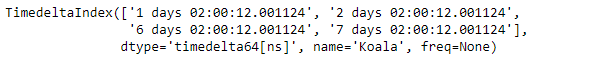As we can see in the output, the `TimedeltaIndex.symmetric_difference()` function has returned a new object which contains only those elements which are not common to both the objects.

My Personal Notes arrow_drop_upCheck out this Author's contributed articles.

If you like GeeksforGeeks and would like to contribute, you can also write an article using contribute.geeksforgeeks.org or mail your article to contribute@geeksforgeeks.org. See your article appearing on the GeeksforGeeks main page and help other Geeks.

Please Improve this article if you find anything incorrect by clicking on the "Improve Article" button below.

Article Tags :

Be the First to upvote.

Please write to us at contribute@geeksforgeeks.org to report any issue with the above content.